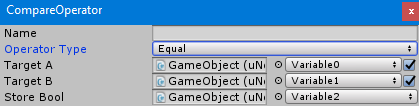# CompareOperatorCompareOperator is used to compare an object to another object and store the result to a variable.

There are 6 operator type to chose:

• Equal used to compare if “Target A” and Target B is equal
• Not Equal used to compare if Target A and Target B is not equal
• Less Than used to compare if Target A is less than Target B
• Greater Than used to compare if Target A is greater than Target B
• Less Than Or Equal used to compare if Target A is less than or equal Target B
• Greater Than Or Equal used to compare if Target A is greater than or equal Target B